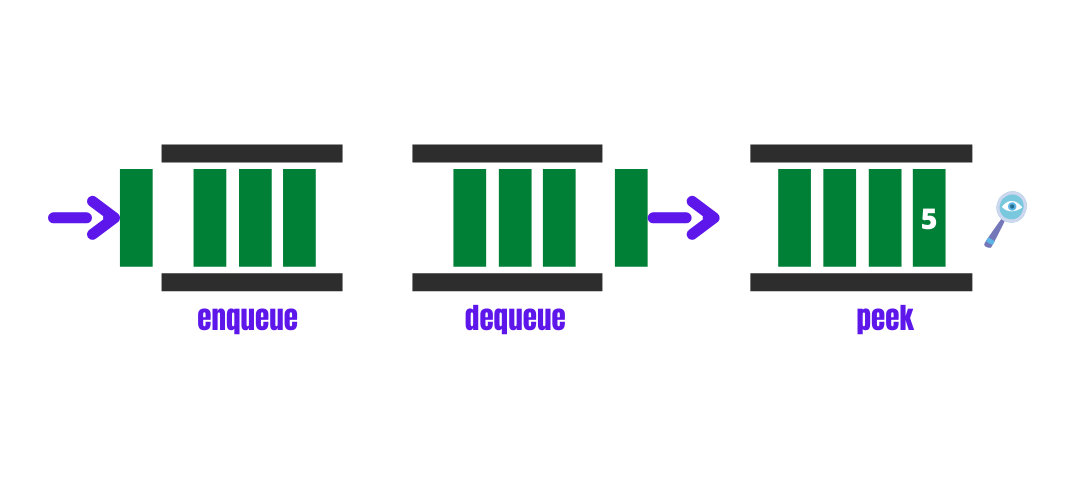# Getting Started with Data Structures - Queue

##### Queues are one of the non-primitive linear data structures very similar to stacks.Batoi Research Group Mar 23, 2021

#### What are queues?

Queues are one of the non-primitive linear data structures very similar to stacks. It follows the principle of first in first out (FIFO) or last in last out (LILO).

#### Working

Queues are containers with two openings that allow them to perform operations like enqueue, dequeue, peek etc. You can understand the queue from a real-world example as a series of people lining up to get on a bus at a bus stop. The first person that gets in the line gets on the bus first.#### Implementation of a Queue Using Arrays

Let’s design our queue using arrays that follow the requirements above.

//C++class Queue{ public:      int arr;     int front,rear;     int size;     Queue(){         front = 0;        rear = 0;        size = 1000;     }      void enqueue(int x){          if(rear == size) cout<<"Queue is full\n";        else{        	arr[rear++] = x;         	cout<<"pushed "<        }    }     void dequeue(){         if(front == rear) cout<<"Queue is empty\n";         else{        	for(int i=0;i        		arr[i] = arr[i+1];        }        rear--;    }      int peek(){         if(front == rear) {        	cout<<"Queue is empty\n";  return INT_MIN;    	}          return arr[front];     }    bool isEmpty(){    	return front == rear;    }};

As we have implemented a queue using arrays, the size of the queue is not dynamic; also, the dequeue operation takes O(n) time which is not good. Every time we do an enqueue, dequeue or peek operation to ensure that the queue is not full or empty; otherwise, we might receive garbage values.

Time complexities:

enqueue= O(1)dequeue =  O(n)peek = O(1)

#### Implementation of a Queue Using Linked Lists

Let’s design a queue using linked lists that follows the requirements above.

class Node{     public:     int val;     Node* next;     Node(int data){         val = data;         next = NULL;     } }; class Queue{     Node *front,*rear;     public:     Queue(){     rear = front = NULL;     }     void enqueue(int x){         Node* newNode = new Node(x);         if(front == NULL){            front = newNode;            rear = newNode;        }        else{            rear->next = newNode;            rear = rear->next;        }    cout<<"pushed "<} void dequeue(){     if(front == NULL) cout<<"Queue is empty\n";     else {         Node* temp = front;        front = front->next;        if(front == NULL)        rear = NULL;         delete(temp);        cout<<"removed the front element\n";     } }  int peek(){     if(!front){         cout<<"Queue is empty\n";         return INT_MIN;         }     return front->val;     }    bool isEmpty(){    return front == NULL;    }};

Implementing a queue using linked lists instead of arrays makes it dynamic in size. The time complexity of the dequeue operation is also improved.

Time complexities:

enqueue = O(1)dequeue = O(1)peek = O(1)

#### C++ STL Queue

C++ Standard template library provides in-built queue containers.

#include #include  using namespace std;int main() {    queue q;    for(int i=0;i<50;++i){        q.push(i);    }    while(!q.empty()){        cout<";        q.pop();    }     return 0;}

#### Types of the Queue

Double-ended queue: In this type of queue, unlike the conventional queue, data can be inserted from both ends and removed from both ends.

Input restricted queue: This is a special case of a double-ended queue where data can be inserted from only one end but can be removed from both ends.

Output restricted queue: This is a special case of a double-ended queue where data can be inserted from both ends but can be removed from only one end.

Priority queue: This is a special type of queue that maintains the order based on priority rather than what is inserted first. For example, A priority queue can hold the greatest number among all the numbers in the queue in front. Every time the queue is popped, the queue rearranges itself such that the largest element among the elements of the queue is still in the front.

#### Uses of the Queue

CPU Scheduling: When multiple processes require CPU at the same time, various CPU scheduling algorithms are used, which are implemented using the Queue data structure

Breadth-first search: Queue is a data structure used to traverse a graph/tree in a Breadth-first order.

Recognising palindrome

Handling Interrupts: The processor handles interrupts in the order they are triggered. First come, first served.

Print spooling: Documents are loaded in a buffer, and then the printer prints the documents in order. In this way, you don’t have to wait for one printing job to be finished.

c

### Need our assistance? We are available with you.